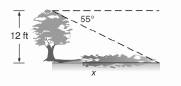Chapter 11.CR, Problem 21CR### Elementary Geometry for College St...

6th Edition
Daniel C. Alexander + 1 other
ISBN: 9781285195698

#### Solutions

Chapter
Section### Elementary Geometry for College St...

6th Edition
Daniel C. Alexander + 1 other
ISBN: 9781285195698
Textbook Problem
1 views

# In exercises 21 to 30. Use the drawings. Where provided, to solve each problem. Angle measures should be found to the nearest degree; lengths should be found to the nearest tenth of a unit.In the evening, a tree that stands 12 ft tall casts a shadow. If the angle of depression from the top of the tree to the tip of the shadow is 55 ° , what is the length of the shadow?To determine

To find:

what is the length of the shadow.

Explanation

Procedure used:

In any right triangle ABC with mA=θ we have the following ratios.

sinθ=OppositeHypotenuse

Calculation:

Given:

Height of the tree =12 ft

Angle of depression of the shadow =55°

Let us structure the given figure as follows as a right triangle.

In the above figure CZ is parallel to AB and CA is the transversal.

Thus, by the property that the alternate interior angles are equal, we have

mZCA=mCAB=55°

Thus, in ABC

mA=55°

BC=12 ft (Height of the tree)

(i

### Still sussing out bartleby?

Check out a sample textbook solution.

See a sample solution

#### The Solution to Your Study Problems

Bartleby provides explanations to thousands of textbook problems written by our experts, many with advanced degrees!

Get Started

#### Explain what happens during each of the two stages of the two-factor ANOVA.

Essentials of Statistics for The Behavioral Sciences (MindTap Course List)

#### Expand each expression in Exercises 122. (x+1x)2

Finite Mathematics and Applied Calculus (MindTap Course List)

#### In Exercises 1124, find the indicated limits, if they exist. 14. limx3x3x+4

Applied Calculus for the Managerial, Life, and Social Sciences: A Brief Approach

#### True or False: xy + 6x2y = 10 x3 is linear.

Study Guide for Stewart's Single Variable Calculus: Early Transcendentals, 8th